• VB 用过程求组合，输入m和n，按等号键可得组合，来看如下的简单代码： 　Private Sub f(m As Integer, p As Long) '自定义通用过程，用于求阶乘 　Dim i As Integer '声明局部变量 　p = 1 '赋初值 　For i = 1 ...
• VB生成不重复的随机数 我的建议是：第一步、先做一数组，存上这35个数（可以不是连续的，也可以是人名、字符串什么的）；...重复到从第35-35个数之间随机抽取一个数，这时直接输出最后一个就行了。
• VB程序设计练习题（一）

千次阅读 2021-05-09 13:48:34
VB程序设计练习题（） 文章目录 目录 前言 内容 1、十进制转二进制 2、程序实现功能：小明过年要给他的40位同学各寄一张贺卡，贺卡的价格有3元、2元和1元三种，他一共有100元，怎样购买正好把钱用完。把...

文章目录

目录

VB程序设计练习题（一）

文章目录

前言

内容

1、十进制转二进制数

2、购买贺卡问题

三、用滚动条改变标签颜色

4.猴子吃桃，递推举法

5、比赛最高分与最低分

6、人口问题

7.二维数组矩阵输出

8.二维数组4*4矩阵输出

9、（二维数组）求二维数组四周元素的和

10.输出5*5矩阵求对角线之和

11、输出三角形

12.单击窗体，利用双重循环输出如下的图案。

13、输出连续和为1250的正整数范围

14、求Π的值

15、单词首字母大写

16、辗转相除法求最大公约数

17、累乘与累加

18、双胞胎数

19、for语句输出三角形

20、杨辉三角形

前言

备考技能考的一些陌生的VB练习题

难度比较大

有什么问题可以在评论区发表

内容

1、十进制转二进制数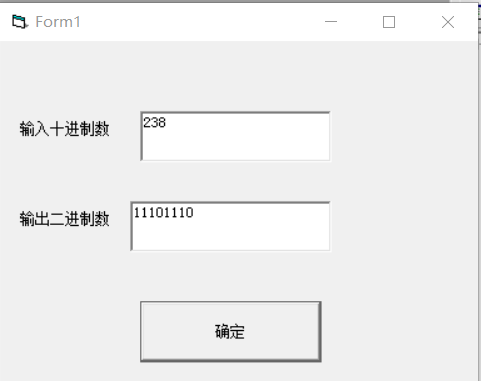答案:

Private Sub Command1_Click()

Dim i As Integer

a = Val(Text1.Text)

If a >= 0 And a <= 255 Then

For i = 1 To 8

If a Mod 2 = 1 Then

a = a \ 2

b = b & "1"

Else

a = a / 2

b = b & "0"

End If

Next

Else

MsgBox "请输入正确的十进制数："

End If

Text2.Text = StrReverse(b)

End Sub

2、购买贺卡问题

程序实现功能：小明过年要给他的40位同学各寄一张贺卡，贺卡的价格有3元、2元和1元三种，他一共有100元，怎样购买正好把钱用完。把所有可能的组合显示出来。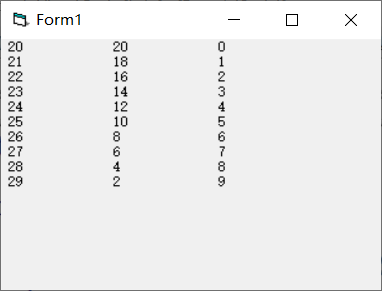答案：

Private Sub Form_Click()

dim a,b,c,d,e,f

a = 3

b = 2

c = 1

For d = 0 To 34

For e = 0 To 50

For f = 0 To 100

If a * d + b * e + c * f = 100 And d + e + f = 40 Then

Print d; Space(5); e; Space(5); f

End If

Next

Next

End Sub

三、用滚动条改变标签颜色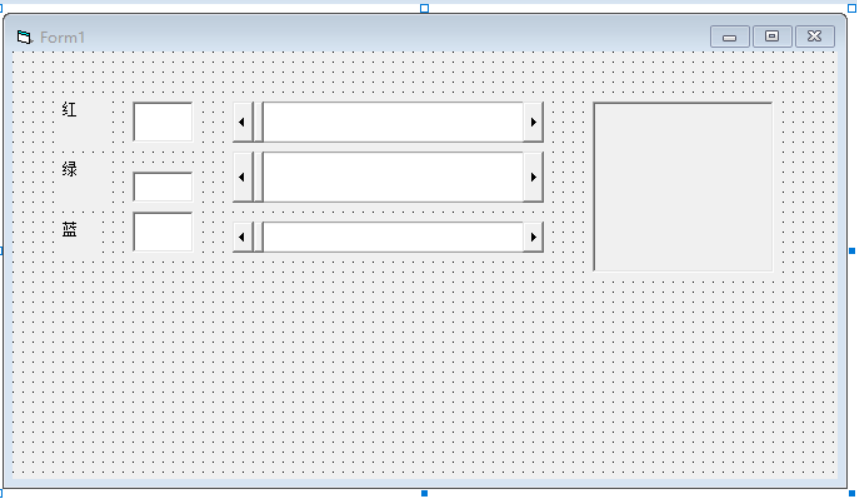答案:

Private Sub HSB_Change()

Text3.Text = HSB.Value

Label4.BackColor = RGB(Val(Text1.Text), Val(Text2.Text), Val(Text3.Text))

End Sub

Private Sub HSB_Scroll()

Text3.Text = HSB.Value

Label4.BackColor = RGB(Val(Text1.Text), Val(Text2.Text), Val(Text3.Text))

End Sub

Private Sub HSG_Change()

Text2.Text = HSG.Value

Label4.BackColor = RGB(Val(Text1.Text), Val(Text2.Text), Val(Text3.Text))

End Sub

Private Sub HSG_Scroll()

Text2.Text = HSG.Value

Label4.BackColor = RGB(Val(Text1.Text), Val(Text2.Text), Val(Text3.Text))

End Sub

Private Sub HSR_Change()

Text1.Text = HSR.Value

Label4.BackColor = RGB(Val(Text1.Text), Val(Text2.Text), Val(Text3.Text))

End Sub

Private Sub HSR_Scroll()

Text1.Text = HSR.Value

Label4.BackColor = RGB(Val(Text1.Text), Val(Text2.Text), Val(Text3.Text))

End Sub

4.猴子吃桃，递推举法

要以上一个本身做铺垫才能求出下一个的值的，如果把i设为循环值，在循环中的i只是它的下标签，在循环中不需要把i当系数，也就是说循环中不出现i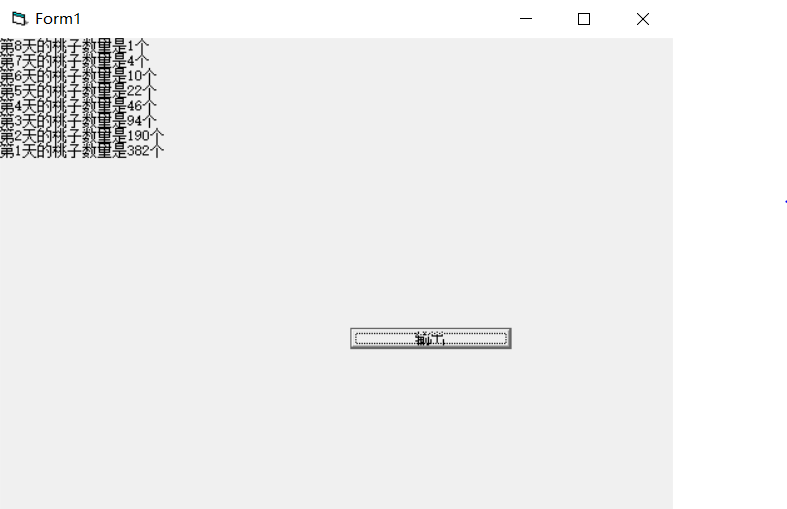答案:

Private Sub Command1_Click()

x = 1

Print "第8天的桃子数量是1个"

For i = 7 To 1 Step -1

x = (x + 1) * 2         #循环中不需要出现i

Print "第" & i & "天的桃子数量是" & x & "个"

Next

End Sub

5、比赛最高分与最低分

某次歌曲大奖赛，有7个评委对某个参赛者的打分数，按照去掉一个最高分和最低分的计算办法，求出该参赛者的平均得分以及最高分和最低分。

答案：

Private Sub Command1_Click()

Dim a(1 To 7), i, s, b As Integer

s = 0

For i = 1 To 7      ‘先定义ifor循环，用对话框的方式输入每个评委的得分。

a(i) = Val(InputBox("第" & i & "个评委得分"))

s = s + a(i)         ‘把所有评委的得分加起来

Next

Min = a(1)          ‘先定义Min等于a(1)的值

For i = 1 To 7

If a(i) > Max Then     ‘让每个数都于Max比较，若大于则替换值。（Max初始最为0

Max = a(i)

End If

Next

For i = 1 To 7          ‘让每个数都于Min比较，若小于则替换值。（Min=a1））

If a(i) < Min Then

Min = a(i)

End If

Next

s = s - (Max + Min)        ‘最后减掉最大值与最小值

b = s / 5                  ‘求平均值

Print "最大值" & Max & vbCrLf & "最小值" & Min & vbCrLf & "去掉最高分与最低分的平均值是" & b     ‘输出

End Sub

6、人口问题

我国目前有13亿的人口，按人口年增长率0.8%计算，多少年后我国人口将超过26亿

答案：

Private Sub Command1_Click()

a = 13      ‘先定义a为13

s = 0

Do While a <= 26

a = a * (1 + 0.008)

s = s + 1

Loop

Print s

End Sub

7.二维数组矩阵输出

（二维数组）随机生成一个整型的二维数组，范围在[10,20]之间，以下三角形形式输出该数组。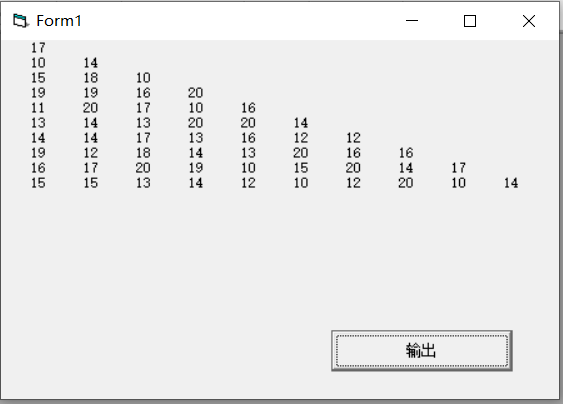答案：

Private Sub Command1_Click()

Dim a(1 To 10, 1 To 10) As Integer

For i = 1 To 10

For j = 1 To 10

a(i, j) = Int(Rnd * (20 - 10 + 1) + 10)

Next

Next

For i = 1 To 10

For j = 1 To i

Print Spc(3); a(i, j);

Next

Print

Next

End Sub

8.二维数组4*4矩阵输出

（二维数组）利用随机函数生成一个4*4的矩阵（即二维数组），范围是[20,50]内的整数，输出该矩阵所有数据的之和。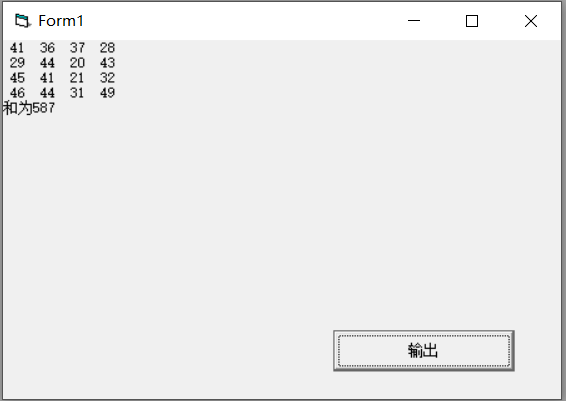答案：

Private Sub Command1_Click()

Dim a(1 To 4, 1 To 4) As Integer

For i = 1 To 4

For j = 1 To 4

a(i, j) = Int(Rnd * (50 - 20 + 1) + 20)

Print a(i, j);

s = s + a(i, j)

Next

Print

Next

Print "和为" & s

End Sub

9、（二维数组）求二维数组四周元素的和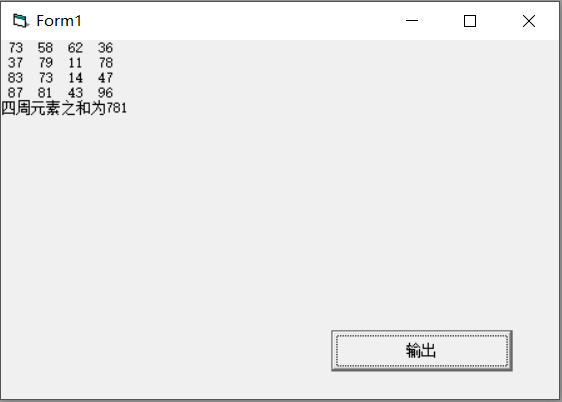答案：

Dim a(4, 4)
Private Sub Command1_Click()
For i = 1 To 4

For j = 1 To 4

If i = 1 Or j = 1 Or i = 4 Or j = 4 Then

Sum = Sum + a(i, j)

End If

Next

Next

Print "四周元素之和为" & Sum

End Sub

Private Sub Form_Click()
For i = 1 To 4
For j = 1 To 4
a(i, j) = Int(Rnd * (99 - 10 + 1) + 10)
Print a(i, j);

Next
Print

Next

End Sub

10.输出5*5矩阵求对角线之和

(二维数组)用二维数组加双重循环输出5*5的方阵，每个数组的值的区间[10,99]之间，并且输出对角线的值的之和。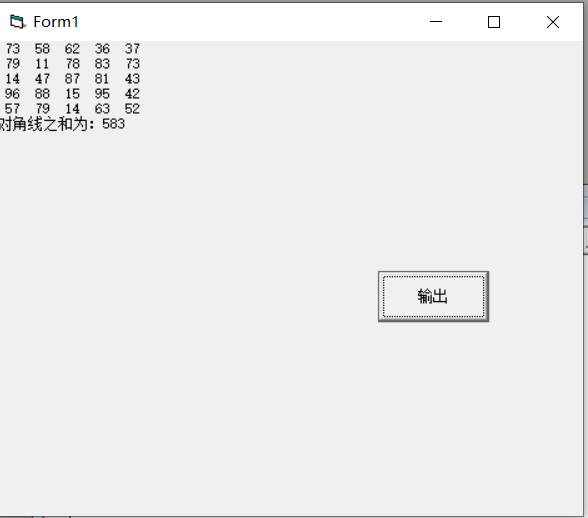答案：

Private Sub Command1_Click()

Dim a(1 To 5, 1 To 5) As Integer

For i = 1 To 5

For j = 1 To 5

a(i, j) = Int(Rnd * (99 - 10 + 1) + 10)

If i = j Then

s = s + a(i, j)

End If

If i + j = 6 Then

b = b + a(i, j)

End If

Print a(i, j);

Next

Print

Next

Print "对角线之和为：" s + b - a(3, 3)

End Sub

11、输出三角形

利用双重循环输出以下的图案。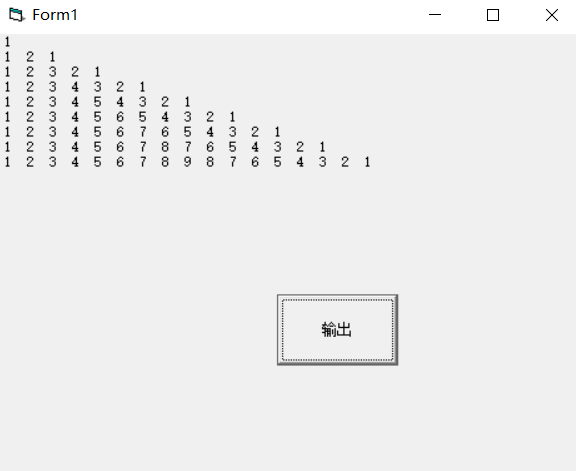答案：

Private Sub Command1_Click()

For i = 1 To 9

For j = 1 To I         以上是先输出一个直角三角形的1i的数。（循环会先把上面的j

Print j;              循环全部输出出来再输出下一个循环，即e

Next

If i >= 2 Then          这里开始输出e的循环 i-11的数。

For e = i - 1 To 1 Step -1

Print e;

Next

End If

Print

Next

End Sub

12.单击窗体，利用双重循环输出如下的图案。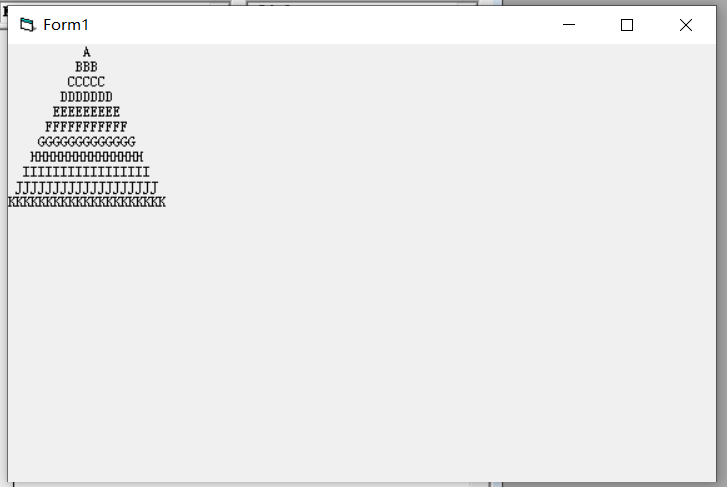答案：

Private Sub Form_Click()

For i = 65 To 75

Print Space(75 - i);

For j = 129 To 2 * i - 1

Print Chr(i);

Next

Print

Next

End Sub

13、输出连续和为1250的正整数范围

在窗体上创建一个命令按钮，标题为“计算并输出”；程序运行后，如果单击命令按钮，程序将计算500以内两个数之间（包括开头和结尾的数）所有连续数的和为1250的正整数，并在窗体上显示出来，这样的数有多组，程序输出每组开头与结尾的正整数，并用“~”连接起来，如图所示。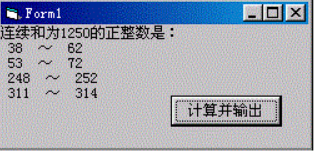答案：

Private Sub Command1_Click()

Print "连续和为1250的正整数是："

For i = 1 To 500   首先定义一个双重循环。I1500ji500，所以j的循环要取

For j = i To 500    决于i

s = s + j           累加

If s > 1250 Then   看看累加的s大于1250吗，若大于则停止j循环，接着i循环。

Exit For

ElseIf s = 1250 Then 若等于s后，则输出i循环所用的值，也就是开头，再输出j循环的值，

Print i; "~"; j        也就是结尾。

Exit For           这里还是要停止循环，

End If

Next

s = 0            s=0，初始化，让下一次循环用

Next

End Sub

14、求Π的值

布局如下图。单击按钮，根据下面的公式计算Π的值：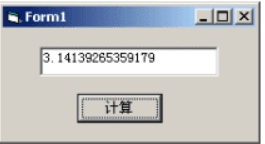Π/4=1-1/3+1/5-1/7+…….1/10001

用do….while 实现程序。

答案：

Private Sub Command1_Click()

Dim r , s, i

r = 0

s = 1

i = 1

Do While 1 / i >= 0.0001

r = r + s / i

i = i + 2

s = -s

Loop

Text1.Text = r * 4

End Sub

15、单词首字母大写

按照如下图布置，程序运行后，单击按钮，文本框中的每个单词的首字母变成大写。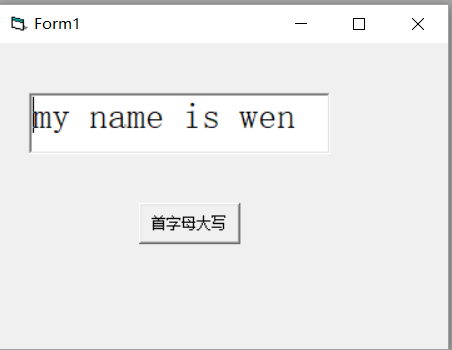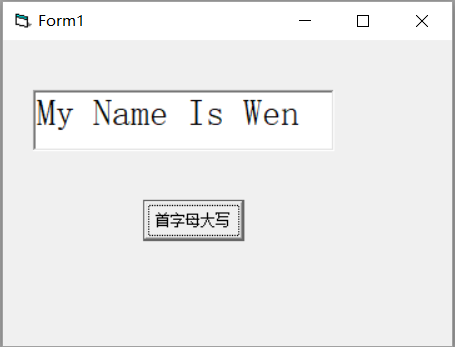答案：

Private Sub Command1_Click()

Dim i, a, f, c

f = 0                                首先定义f=0c=””

c = ""

For i = 1 To Len(Text1.Text)              循环i=1text1.text的长度

a = Mid(Text1.Text, i, 1)                 a=循环等于每个字母

If i = 1 Then                          当第一个字母的时，第一个字母转化为大写

a = UCase(a)

End If

If f = 1 Then                         f=1时，这里只有运行下面那个a=” ”，才能触发

a = UCase(a)                         当运行下面的a=” “的时候，f才会为1。也就是说

f = 0                                空格的后一项字母为大写。

End If

If a = " " Then

f = 1

End If

c = c & a

Next

Text1.Text = c

End Sub

16、辗转相除法求最大公约数

创建3个文本框，计算text1和text2的正整数的最大公约数，并显示在text3中。

（要求使用do…….loop语句来实现）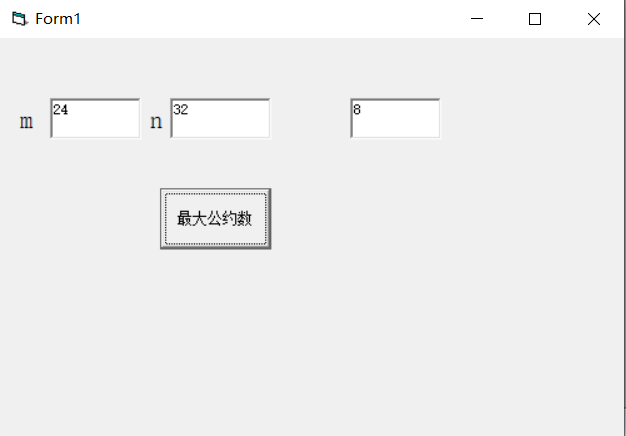答案：

Private Sub Command1_Click()

m = Val(Text1.Text)

n = Val(Text2.Text)

If n > m Then
t = n
n = m
m = t
End If

Do

r = m Mod n

If r = 0 Then Exit Do

m = n

n = r

Loop

Text3.Text = n

End Sub

17、累乘与累加

点击窗体，已知s=1+1/3!+1/5!+1/7!+1/9!.将s的结果输出到窗体上。使用do while….loop语句完成程序。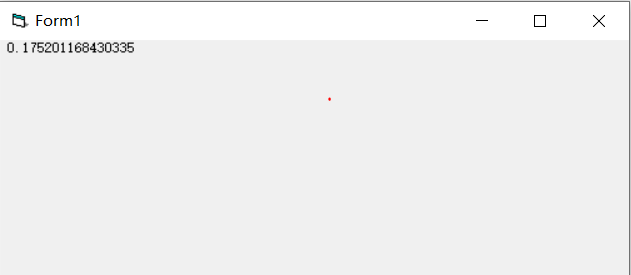答案：

Private Sub Form_Click()

Dim i As Integer

i = 1           这相当于for的初始值

s = 1

Do While i <= 9  这相当于for的结束值

i = i + 1         公差，相当于for循环里的step（必须定义）

s = s * i

If i Mod 2 = 1 Then

p = p + 1 / s

End If

Loop          这里相对于next

Print p

End Sub

18、双胞胎数

若两个素数之差为2，则称这两个素数为双胞胎数。求出[200，1000]之内有多少对双胞胎数。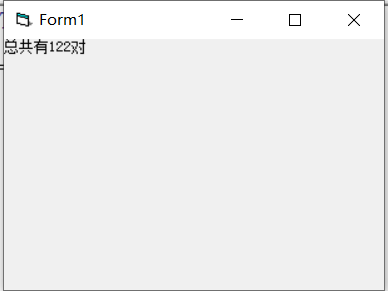答案：

Private Sub Form_Click()

For i = 200 To 1000

f = 0

For j = 2 To i - 1

If i Mod j = 0 Then

f = 1

Exit For

Else

f = 0

End If

Next

If f = 0 Then            1.当求出2001000之间第一个素数时（从小到大），就循环下

For e = 200 To 1000        组素数。

n = 0

For a = 2 To e - 1

If e Mod a = 0 Then

n = 1

Exit For

Else

n = 0

End If

Next

If f = 0 Then        2.当是素数时

If i + 2 = e Then .则判断第一组中的素数是否加2等于第二组中的素数。（相差为2

s = s + 1  ，若满足则s1.

Exit For  ，退出循环（因为已经找到了，就不可能有第二个一样的）

End If

End If

Next

End If

Next

Print "总共有" & s & "对"

End Sub

19、for语句输出三角形

（新思路）用for语句输出如下图案。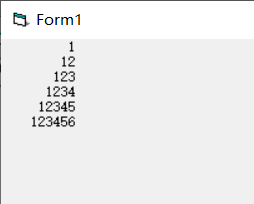答案：

Private Sub Command1_Click()

Dim i, j As Integer

a = "123456"            先定义一个a字符串是16

For i = 1 To 6

Print Space(10 - i); Mid(a, 1, i)  利用mid函数来选择

Next

End Sub

20、杨辉三角形

编写适当的事件过程，在窗体上输出相对应行数的杨辉三角形，点击窗体弹出inputbox对话框，在对话框中输入行数，打印出如下图所示的杨辉三角形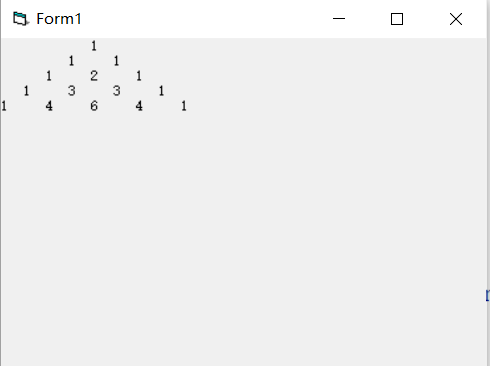答案：

Private Sub Form_Click()

Dim i As Integer, j As Integer

Dim a As Integer

a = Val(InputBox("请输入行数"))

Dim c(9, 9) As Integer

If a > 0 Then

For i = 1 To a

Print Space(3 * a - 3 * i);

For j = 1 To i

If i = 1 Or j = i Then       ‘当为三角形两边的时候，输出1

c(i, j) = 1

End If

If i = 2 Or j = i - 1 Then              ‘当为三角形的两边里面一个的时候输出行数-1

c(i, j) = i - 1

End If

If i > 2 And j < i - 1 Then                    否则都以自身上面一行对角线两个数的和。

c(i, j) = c(i - 1, j - 1) + c(i - 1, j)

End If

Print c(i, j) & "     ";                       ‘输出

Next

Print

Next

End If

End Sub

点赞、关注

展开全文程序设计 vb6 编程语言
• VB6.0升级到VB.NET后，发现随机数函数也发生了变化，在VB.NET中Random类是种能够产生满足某些随机性统计需求的数字序列的伪随机生成器。 在代码把光标定位到单词Random，点F1键获取帮助。 、Random类常用...

VB6.0升级到VB.NET后，发现随机数函数也发生了变化，在VB.NET中Random类是一种能够产生满足某些随机性统计需求的数字序列的伪随机数生成器。
在代码把光标定位到单词Random，点F1键获取帮助。
一、Random类常用知识点（以下内容来源于帮助，点击进入Random类帮助网页）：
1、构造函数：
（1）、Random()
使用与时间相关的默认种子值，初始化 Random 类的新实例。
（2）、Random(Int32)
使用指定的种子值初始化 Random 类的新实例。
参数为种子值，数据类型为Int32，用来计算伪随机数序列起始值的数字。 如果指定的是负数，则使用其绝对值。
2、方法：
（1）、Next：返回一个随机整数。
A、无参数：Next()——返回一个非负随机整数。
B、一个参数：Next(maxValue) ——返回一个小于所指定最大值的非负随机整数。
参数（maxValue）：类型为Int32，要生成的随机数的上限（随机数不能取该上限值）。maxValue 必须大于或等于 0。
返回值：类型为Int32，大于或等于零且小于 maxValue 的 32 位有符号整数，即：返回值的范围通常包括零但不包括 maxValue。 但是，如果 maxValue 等于 0，则返回 maxValue。
C、两个参数：Next(minValue,maxValue)——返回在指定范围内的任意整数。
参数（minValue）：类型为Int32，返回的随机数的下界（随机数可取该下界值）。
参数（maxValue）：类型为Int32，返回的随机数的上界（随机数不能取该上界值）。 maxValue 必须大于或等于 minValue。
返回值：类型为Int32，一个大于等于 minValue 且小于 maxValue 的 32 位带符号整数，即：返回的值范围包括 minValue 但不包括 maxValue。 如果 minValue 等于 maxValue，则返回 minValue。
（2）、NextBytes：用随机数填充指定字节数组的元素。
NextBytes(buffer)
参数（buffer）：Byte[]，包含随机数的字节数组。
说明：字节数组的每个元素设置为随机数字大于或等于 0，且小于或等于MaxValue。
（3）、NextDouble：返回一个大于或等于 0.0 且小于 1.0 的随机双精度型浮点数。
没有参数，返回值类型为Double。
3、使用方法：
要生成各种类型的随机数，必须先得到它的实例对象，然后再生成随机数。注意只实例化一个对象，可以多次方法调用，生成的数字分布均匀，每个数字返回的可能性均相等。
二、Random类生成随机数的弱点
Random类生成随机数是伪随机数，所以在Random的内部产生机制中还是有一定规律的，并非是真正意义上的完全随机。 如果种子值相同则每次均产生相同的随机数。如下面代码在构造函数中指定了种子值：

Dim rand As Random = New Random(66)
For i As Integer = 0 To 10
Me.Label1.Text = Me.Label1.Text & rand.Next() & Environment.NewLine
Next
End Sub

每次运行程序均生成相同的随机数序列，如下图所示，不信你就测试一下。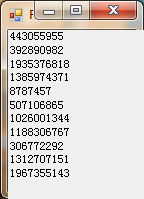三、怎样才能每次运行程序时均生成不同的随机数？
其实很简单，就是每次运行程序时改变Random类的构造函数的种子值，可以有以下做法：
第一种方式： 使用无参数的Random()构造函数；
默认种子值是使用DateTime.Now.Ticks作为种子值的，如果触发Random函数间隔时间很短， 就有可能造成产生一样的随机数。
第二种方式： 使用RNGCryptoServiceProvider生成种子值；生成速度较慢。
在帮助里有看到推荐，如下图：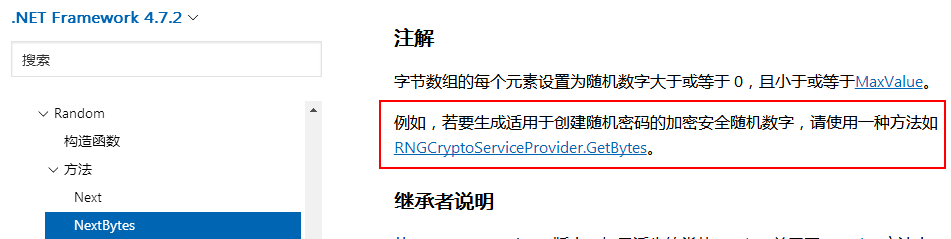第三种方式： 使用Guid生成种子值； 生成速度快。
测试代码如下：

Public Class Form1
''' <summary>
''' 通过RNGCryptoServiceProvider获取种子值
''' </summary>
''' <returns></returns>
Private Shared Function GetRandomSeed() As Integer
Dim bytes As Byte() = New Byte(3) {}
Dim rng As System.Security.Cryptography.RNGCryptoServiceProvider = New System.Security.Cryptography.RNGCryptoServiceProvider()
rng.GetBytes(bytes)
Return BitConverter.ToInt32(bytes, 0)
End Function

''' <summary>
''' 通过Guid获取种子值
''' </summary>
''' <returns></returns>
Private Shared Function GetRandomSeedbyGuid() As Integer
'Return New Guid().GetHashCode()
Return Guid.NewGuid().GetHashCode()
End Function

''' <summary>
''' 默认种子值生成随机数
''' </summary>
''' <param name="sender"></param>
''' <param name="e"></param>
Private Sub BtnTime_Click(sender As Object, e As EventArgs) Handles BtnTime.Click
Dim rand As Random = New Random()
Me.Label1.Text = ""
For i As Integer = 0 To 10
Me.Label1.Text = Me.Label1.Text & rand.Next() & Environment.NewLine
Next
End Sub

''' <summary>
''' RNGCryptoServiceProvider种子生成随机数
''' </summary>
''' <param name="sender"></param>
''' <param name="e"></param>
Private Sub BtnRNG_Click(sender As Object, e As EventArgs) Handles BtnRNG.Click
Dim rand As Random = New Random(GetRandomSeed())
Me.Label1.Text = ""
For i As Integer = 0 To 10
Me.Label1.Text = Me.Label1.Text & rand.Next() & Environment.NewLine
Next
End Sub

''' <summary>
''' Guid种子生成随机数
''' </summary>
''' <param name="sender"></param>
''' <param name="e"></param>
Private Sub BtnGuid_Click(sender As Object, e As EventArgs) Handles BtnGuid.Click
Dim rand As Random = New Random(GetRandomSeedbyGuid())
Me.Label1.Text = ""
For i As Integer = 0 To 10
Me.Label1.Text = Me.Label1.Text & rand.Next() & Environment.NewLine
Next
End Sub
End Class

四、生成数字和字母组合的随机数
开始想到用ASCII码，但数字、小写字母及大写字母的ASCII码是不连续的，觉得不是很理想，下面的方法感觉还不错，做个笔记。

Public Function GetAlphanumeric() As String
Dim str As String = "0123456789abcdefghijklmnopqrstuvwxyzABCDEFGHIJKLMNOPQRSTUVWXYZ"
Dim rnd As New Random()
'返回数字
'Return rnd.[Next](10).ToString()
'返回小写字母
Return str.Substring(10 + rnd.[Next](26), 1)
'返回大写字母
'Return str.Substring(36 + rnd.[Next](26), 1)
'返回大小写字母混合
'Return str.Substring(10 + rnd.[Next](52), 1)
'返回小写字母和数字混合
'Return str.Substring(0 + rnd.[Next](36), 1)
'返回大写字母和数字混合
'Return str.Substring(0 + rnd.[Next](36), 1).ToUpper()
'返回大小写字母和数字混合
'Return str.Substring(0 + rnd.[Next](61), 1)
End Function

五、生成不重复随机数
1、 从N个数中随机取出一个数，与数组第N个数调换；
2、 从前N-1个数中随机取出一个数，与第（N-1）个数调换；
……
代码通过_end-1，把生成过的数用最后一个数代替，省去删除移动的开销。
代码如下：

Public Function GetRandomSequence(ByVal total As Integer) As Integer()
Dim sequence As Integer() = New Integer(total - 1) {}
Dim output As Integer() = New Integer(total - 1) {}

For i As Integer = 0 To total - 1
sequence(i) = i
Next

Dim random As Random = New Random()
Dim _End As Integer = total - 1

For i As Integer = 0 To total - 1
Dim num As Integer = random.[Next](0, _End + 1)
output(i) = sequence(num)
sequence(num) = sequence(_End)
_End -= 1
Next

Return output
End Function

本文参考了以下博文：
不重复随机数列生成算法
学习过程得到网友uruseibest的帮助，表示感谢！

展开全文Random 不重复随机数 随机数种子
• 判断三位数是否为水仙花数

千次阅读 2021-05-22 13:07:01
用C语言写出判断一个三位是否为水仙花的程序请给正确的答案，不懂的别进1.一个主程序，输出所有的水仙花程序，可参考如下： #include int main(int argc, char *argv[]) { int i,n1,n2,n3,k=0; for (i=100;iC...

用C语言写出判断一个三位数是否为水仙花数的程序

请给正确的答案，不懂的别进

1.一个主程序，输出所有的水仙花数程序，可参考如下： #include int main(int argc, char *argv[]) { int i,n1,n2,n3,k=0; for (i=100;i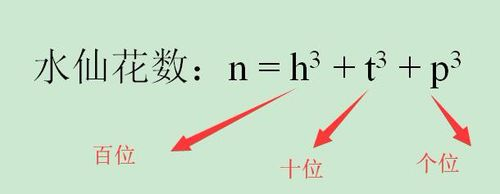C语言编程输入一个三位正整数判断是否为”水仙花数”//提示：153 370 371 407 是水仙花数 #include void main() { int i,j,k,n; printf("请输入一个三位整数\n"); scanf("%d",&n); i=n/100; /*分解出百位*/ j=n/10%10; /*分解出十位*/ k=n%10; /*分解出个位*/ if(i*100+j*10+k==i*i*i+j*j*j+k*k*k)

功能：调用函数fun判断一个三位数是否"水仙花数"。

功能：调用函数fun判断一个三位数是否"水仙花数"。 在main函数中从键盘int fun(int n) { int a,b,c; a=n%10; b=n/10%10; c=n/100; if(a*a*a+b*b*b+c*c*c==n) return 1; else return 0; }

Python输入一个三位数，判断其是否为水仙花数，所###水仙花数适用于任何位数的整数while True: a=int(input("输入一个整数：")) bb=0 b=a while b。=0: bb+=(b%10)**3 ##取余数CSS布局HTML小编今天和大家分享三次方 b = b // 10 ##取相除后的整数部分 if bb==a: ##判断两者是否相等 print("输入为水仙花数：",a) else: print

c语言。。用if语句编程，输入一个三位数，判断是否三位的水仙花数就是每一位的立方之和和这个三位数相等的数，用if语句编程，输入一个三位数，判断是否为水仙花数，可以参考以下的代码： include main() { int x,a,b,c; scanf("%d",x); a=x/100; b=(x/10)%10; c=x%10; if(x==a*a*a+b*b*b+c*c*c)

vb编程，输入一个三位数,判断是否为水仙数

(水仙数的特点：个位数、十位数和百位数的立方和等于该数自身的数)

Private Sub Command1_Click() Dim a As Integer, b As Integer, c As Integer, s As Integer s = Text1.Text a = s \ 100 b = (s - 100 * a) \ 10 c = s - 100 * a - 10 * b If a ^ 3 + b ^ 3 + c ^ 3 = s Then MsgBox s & "是水仙花数" Else M

java5.从键盘输入一个三位数，并判断是否是水仙花水仙花数, 主要用到的是基础的运算符相关的知识 参考代码和注释 import java.util.Scanner;public class Demo {static Scanner input;public static void main(String[] args) {input = new Scanner(System.in);// int num = Integer.parseInt(i

c语言 输入一个三位数，判断是否是一个“水仙花数”c语言 输入一个三位数，判断是否是一个“水仙花数”，水仙花数是指3位数的#include int sxh(int number);//sxh=水仙花数 int main() { int i; printf("输出一个三位数:"); scanf("%d",&i); if(sxh(i)) printf("the data %5d is yes\n",i); printf("\n"); return 0; } int sxh(int number) //定义判断水仙花数的函数 { i

C语言：写函数判断一个三位数是不是水仙花数，是输最好运用新手入门的代码

#include void main(){int a,b,c,n;printf("请输入一个三位数: ");scanf("%d",&n);a=n%10;b=(n%100-n%10)/10;c=(n-n%100)/100;if(a*a*a+b*b*b+c*c*c==n)printf("%d是水仙花数。",n);else printf("%d不是水仙花数。

判断一个三位数是否是水仙花数，可是不管输入什么水仙花数：是指一个 n 位数 ( n≥3 )，它的每个位上的数字的 n 次幂之和等于它本身。(例如：1^3 + 5^3+ 3^3 = 153) 你可以记一下：三位的水仙花数共有5个：153，370，371，407,417 main() { int i,j,k,n; printf("'water flower'number is:");

展开全文• 整体思路有点像映射的关系，如果存在对应元素的比它第一个大的，就在一个数组对应位置存在这大值，具体思路如下： 利用栈，从左到右依次压入数组中元素的索引，每次判断待压入的下一个元素是否大于占栈顶索引...

解题思路：

整体思路有点像映射的关系，如果存在对应元素的比它第一个大的数，就在一个数组对应位置存在这个大值，具体思路如下：

利用栈，从左到右依次压入数组中元素的索引，每次判断待压入的下一个元素是否大于占栈顶索引对应的元素，若大于，就将元素存到一个新的数组res中，将栈顶索引出栈，对应位置就是栈中刚刚出栈的索引，若小于就压入栈中。最后判断一下剩余的元素，没有大于它的就在res索引位置置-1.

代码实现：

public class Test{
public static int[]findMax(int []arry){
int len=arry.length;
int i=0;
int []res=new int[len];
Stack<Integer> sk=new Stack<Integer>();
while(i<len){
if(sk.isEmpty()||arry[i]<arry[sk.peek()]){
sk.push(i);
i++;
}else{
res[sk.pop()]=arry[i];
}
}
while(!sk.isEmpty()){
res[sk.pop()]=-1;
}
return res;
}
public static void main(String[] args) throws IOException {
// TODO Auto-generated method stub
int array[]=new int[] {1,5,3,6,4,8,9,10};
int res[]=findMax(array);
for(int num:res) {
System.out.println(num);
}
}

展开全文• excel VBA获取EXCEL中的行数和列.
• VB.net中金额大写转换

千次阅读 2016-02-28 00:28:36
'最后一位（分）为0时，加上“整” chnNum = chnNum & CST_CAPSTR_TAIL End If Next End If Return chnNum End Function Public Shared Function ConvertToCapsString(ByVal dec As String) As String Dim...
• VB中产生10个不重复的随机数

万次阅读 2013-06-07 18:05:19
我的理解是把你在哪方面不懂的知识学会就是脚踏实地，就比如说用VB产生10随机数，我们看着好像会了，但没有真正地去实现过；或者说我们已经实现了，但没有去总结，当真正要用到的时候，却摸不着头脑，不知如何去...
• VB初步入门

2020-09-20 11:31:04
VB 入门记录（、Visual Basic 界面简介 打开题目进入界面是这样子的 ​ 图 我们的题目信息在这里 ​ 图二 然后当你双击那个产生按钮的时候 ​ ...编程语言
• 本不想再刻意强调VB什么，怕说多了，成了误导。用与不用，其实都无需解释。但，既然在《互联的围城，已春暖花开，我却心向罗盘荒野》中给了大家学习内化的承诺，又在《明比阅历浅，暗拼体力衰，指下讲武德，码上笑...vb6 vba 多线程 指针 面向对象编程
• #include #include #include #include #include #include #include //#include const QString FILE_PATH("./bailamu_48mm_30dB_A.csv"); int main(int argc, char *argv[])  {   QC
• VB.NET 教程_01_基础语法

万次阅读 多人点赞 2018-08-08 00:48:58
VB.Net是种简单，现代，面向对象的计算机编程语言，由微软开发，将.NET Framework和公共语言运行库的强大功能与作为Visual Basic标志的生产力优势相结合。 Visual Basic .NET（VB.NET）是在.NET Framework上...VB.NET
• VB操作wincc脚本心得

千次阅读 2021-02-11 16:04:11
1、Vbs 操作wincc画面脚本总结： 不使用变量单纯的操作界面1.操作某个画面中控件的属性 如 Home1.Pdl(总窗体) 下的 画面窗口2(画面窗口)Sub OnClick(ByVal Item) Dim objCircleDim screennameDim pwnameSet ...
• VB学习记录——数组

万次阅读 多人点赞 2019-07-06 13:05:49
1.定义一个数组，其实就是让系统在内存中分配一个连续的区域，用来存储数组的元素。其格式为：Dim 数组名字 （下界 To 上界） As 类型。 Dim MarkVB(1 To 30) As Integer '定义一个一维数组 上界30 下界是1 共30...
• ////截取最后一个字符“a”后面的3位字符 123a4a4b5b6 a4b //string c = b.Substring(b.LastIndexOf("a"), 3); //TextBox1.Text = c; ////截取最后一个字符“a”后面所有的字符 123a4a4b5b6 a4b5b6 //string c...VB.NET 学习笔记
• VB学习记录

千次阅读 多人点赞 2017-11-25 16:47:56
、什么是VB VB程序设计是要干什么？ 1.添加、设置对象； 2.对对象添加功能。 编程的基本要素 - 对象object - 类class - 属性property - 方法method - 事件event 事件过程的一般格式： ...对象 编程 class
• 求三个整数中的中位数

千次阅读 2018-10-12 17:00:00
比如三整数是 10 12 11，...最后的结果无非是a是中位 或者b是中位 或者c是中位 如果a是中位则，middle=a 那么a是中位满足什么条件呢？ 想不出来没关系，我们可以举例子 a是总位数，要么是这种情...
• 快速排序（QuickSort） ...总是挑最后一个元素为支点（下实现） 选择一随机元素作为支点。 挑选位数作为支点。 在快速排序的关键过程是分区（）。分区目标，给定一数组和X数组作为支点的元素，将X快速排序 算法
• '有序的快速查找(A->Z),非递归法 '函数:NumFind '参数:Myarray Double数组,L 数组的左边界,R 数组右边界.FNumber 要查找的数据. '返回值:找到,则返回下标,否则,返回-1 '例子: Public   ...vbscript string date
• VB编程语言基础知识点总结（转）

万次阅读 多人点赞 2019-04-11 14:30:10
Visual Basic（简称VB）是Microsoft公司开发的种通用的基于对象的程序设计语言，为结构化的、模块化的、面向对象的、包含协助开发环境的事件驱动为机制的可视化程序设计语言。海风教育投诉 海风教育在线辅导0元...
• VB.NET串口通信例子--我的回忆录

万次阅读 多人点赞 2011-11-13 19:16:38
这是我3年前的一个例子，最近翻出来回忆一下。 串口是计算机上种非常通用设备通信的协议。大多数计算机包含两基于RS232的串口，现在配电脑好像只有一个。串口同时也是仪器仪表设备通用的通信协议；很多GPIB兼容...vb.net integer string byte timer
• 摘要：1）VB6如何通过串口发送和接收二进制数据 2）如何次获取组串口数据 正文如下： 1）二进制收发 MSComm1.InBufferCount = 0 '... MSComm1.RThreshold = 1 '收到RThreshold字符时触发事件MSComm1...串口通信 vb6
• 假设变量nPos为坐标点的个数除以四，表示平均的间隔个数，第一点取第一，第二点取第nPos，第三取第nPos*2，第四取最后一个。 　现在是分析这四坐标点的时候了，把这四坐标点连成一字符串。...VB源码 鼠标手势
• c语言 输出10000以内的亲密。...计算a的各因子的算法：用a依次对i(i=1~a/2)进行模运算，若模运算结果等于0，则i为a的一个因子；否则i就不是a的因子。*程序说明与注释#includeint main(){int a,i,b,...
• VB程序破解

千次阅读 2016-04-02 16:03:34
VB程序也是一个非常有趣的东西，你去看VB的主程序，是用Microsoft Visual C++ 5.0编写的，也就是说，其实里面调用的各种命令和控件，都是段VC++的代码。还有VB运行时必须的“库”(虚拟机)：msvbvm50, msvbvm60,api
• VB 中的基本数据类型

万次阅读 2017-06-11 10:12:31
VB基本数据类型 VB 6．O提供的基本数据类型主要有：字符型数据、数值型数据、货币类型、字节类型、布尔类型、日期类型、通用类型、无符号类型、对象类型等9种数据类型。 1．字符型数据 字符型数据(String)用以定义...
• SubCheckNum() iCount=0 iRow=ActiveSheet.Range("s65535").End(xlUp).Row'取最后一行 iCol=ActiveSheet.Range("dz2").End(xlToLeft).Column'取最后一列 MsgBox"i...
• VB函数大全

万次阅读 2012-03-14 15:50:36
VB函数大全  Calendar 常数 可在代码中的任何地方用下列常数代替实际值： 常数 值 描述 vbCalGreg 0 指出使用的是阳历。 vbCalHijri 1 指出使用的是伊斯兰历法。 -----------------------------------...integer string microsoft object
• VB.NET中怎样实现位运算

千次阅读 2014-04-03 19:54:46c语言 VB.NET 位运算...